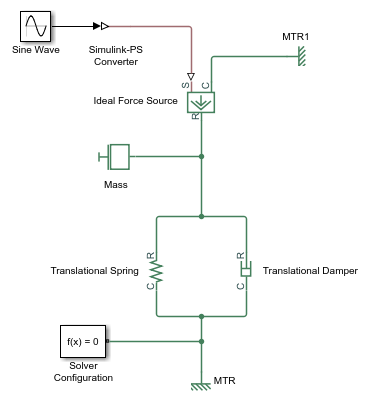# print

Print complete logging tree of Node object

## Syntax

``print(node)``

## Description

example

````print(node)` prints the complete logging tree starting with the specified node. `node` is a `simscape.logging.Node` object. `node` must include a full identifier path to the node, starting with the workspace log variable name. Before you call this object function, you must load the model. You must also have the simulation log variable in your current workspace. Create the simulation log variable by simulating the model with data logging turned on, or load a previously saved variable from a file.```

## Examples

collapse all

Plot complete logging tree for a model, and then a logging tree for a node in the model.

Consider the following model. The model name is `simple_mech2`, and data logging is enabled with the default workspace variable name, `simlog`.Print the complete logging tree for the whole model:

```print(simlog) ```
``` simple_mech2 +-Ideal_Force_Source | +-C | | +-v | +-R | | +-v | +-S | +-f | +-v +-MTR | +-V | | +-v | +-f +-MTR1 | +-V | | +-v | +-f +-Mass | +-M | | +-v | +-f +-Simulink_PS_Converter +-Translational_Damper | +-C | | +-v | +-R | | +-v | +-f | +-v +-Translational_Spring +-C | +-v +-R | +-v +-f +-v +-x```

Print the logging tree just for the Mass block:

```print(simlog.Mass) ```
``` Mass +-M | +-v +-f```

## Input Arguments

collapse all

Initial node for printing the logging tree, specified as a `simscape.logging.Node` object. `node` must include a full identifier path to the node, starting with the workspace log variable name.

Example: `simlog.DC_Motor.Inertia`

## Version History

Introduced in R2010b